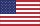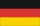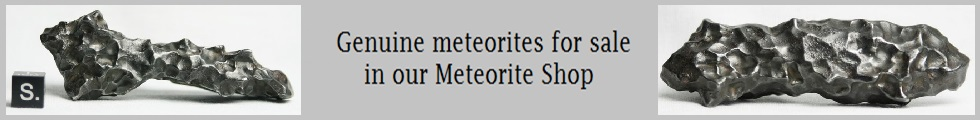English | DeutschThroughout the website we use SI units. Here are the most important conversion units:

 Temperature K (kelvin) 0 K (absolute zero) is -273.15 °C (Celsius) or -459.67 °F (Fahrenheit) 373.16 K (boiling point of water) is 100 °C or 211.73 °F 1 million K ~ 1.8 million °F 1 billion K ~ 1.8 billion °F Mass kg (kilogram) 1 tonne is 1000 kg 1 pound (lb) ~ 0.4536 kg 1 kg = 35.274 ounces (oz) or ~ 32.15075 troy oz 1 solar mass = 1.9891 x 1030 kg Length m (metre) 1 km is 1000 m or 0.62 miles 1 m ~ 3.28 ft (foot) or 39.37 in (inch) 1 AU (Astronomical unit = average distance Earth/Sun) = 149 600 000 km 1 parsec (pc) ~ 3.26 light years or 30.857 x 1012 km or 206 265 AU

All numbers are written according to ISO 31-0. We use the dot as the decimal point (e.g. pi = 3.1415927) and we separate numbers into groups starting with 6 digit numbers. So, up to 99999 there are no separations and starting with 100 000 we separate any number into groups of three digits, such as 750 000 for seven hundred fifty tousand.